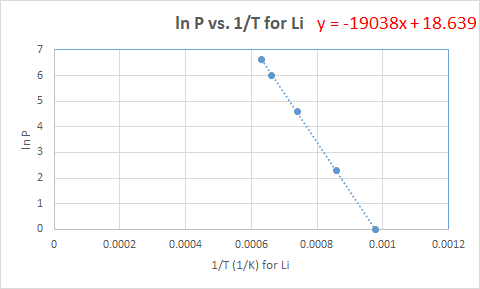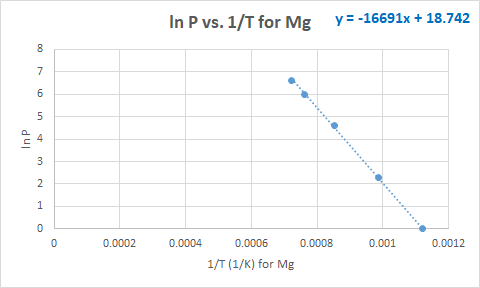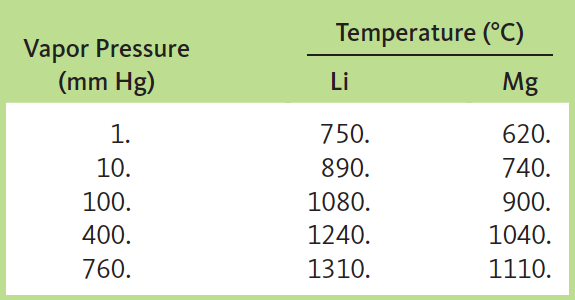# Problem: Plot the following data and determine ΔH vap for magnesium and lithium. In which metal is the bonding stronger?

###### FREE Expert Solution

Clausius-Clapeyron equation:

Higher ΔHvap → stronger bond

Using the given data (convert °C to  K):

 Vapor Pressure ln Pvap T, Li (°C) T, Li (K) 1/T, Li 1 0 750 1023 0.000978 10 2.302585 890 1163 0.00086 100 4.60517 1080 1353 0.000739 400 5.991465 1240 1513 0.000661 760 6.633318 1310 1583 0.000632

 Vapor Pressure ln Pvap T, Mg (°C) T, Mg (K) 1/T, Mg 1 0 620 893 0.00112 10 2.302585 740 1013 0.000987 100 4.60517 900 1173 0.000853 400 5.991465 1040 1313 0.000762 760 6.633318 1110 1383 0.000723

Plot ln P(y-axis) vs. 1/T (x-axis):90% (143 ratings)###### Problem Details

Plot the following data and determine ΔH vap for magnesium and lithium. In which metal is the bonding stronger?Frequently Asked Questions

What scientific concept do you need to know in order to solve this problem?

Our tutors have indicated that to solve this problem you will need to apply the Clausius-Clapeyron Equation concept. You can view video lessons to learn Clausius-Clapeyron Equation. Or if you need more Clausius-Clapeyron Equation practice, you can also practice Clausius-Clapeyron Equation practice problems.

What professor is this problem relevant for?

Based on our data, we think this problem is relevant for Professor Griffith's class at UMD.

What textbook is this problem found in?

Our data indicates that this problem or a close variation was asked in Chemistry: An Atoms First Approach - Zumdahl Atoms 1st 2nd Edition. You can also practice Chemistry: An Atoms First Approach - Zumdahl Atoms 1st 2nd Edition practice problems.